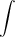# How to solve Green’s Theorem on the Ti89

How do I solve belows Greens Theorem problem using Calculus Made Easy on the TI89 ?

 F · dr.C

F(x, y) =y − cos y, x sin y, C is the circle (x − 6)2 + (y + 2)2 = 16 oriented clockwise

Please do this: select F6 Vector Calculus , F2 8 : and enter as follows

[M,N]= [y-cos(y),x*sin(y)]

solve (x − 6)2 + (y + 2)2 = 16

(y + 2)2 = 16 – (x − 6)2

y = + – sqrt (16 – (x − 6)2) – 2

thus
y1,y2 =[ – sqrt
(16
(x  6)2 )
– 2 ,   sqrt (16(x  6)2 )
– 2 ]

we have a circle centered at [6,2] of radius 4
thus x varies from 2 to 10

x1 , x2 = [2,10]

Voila!!!